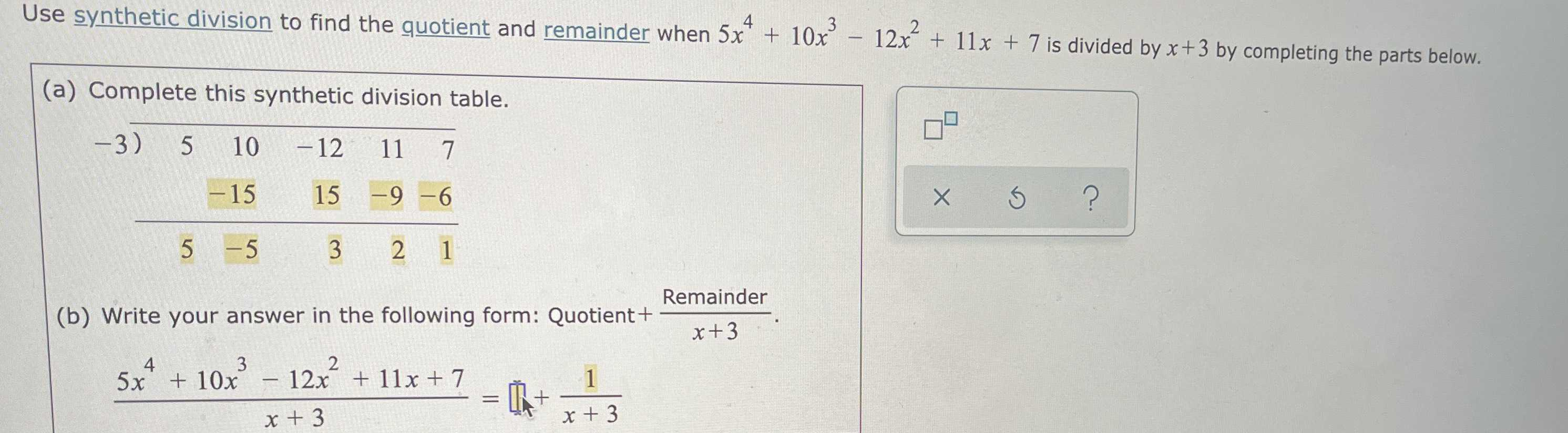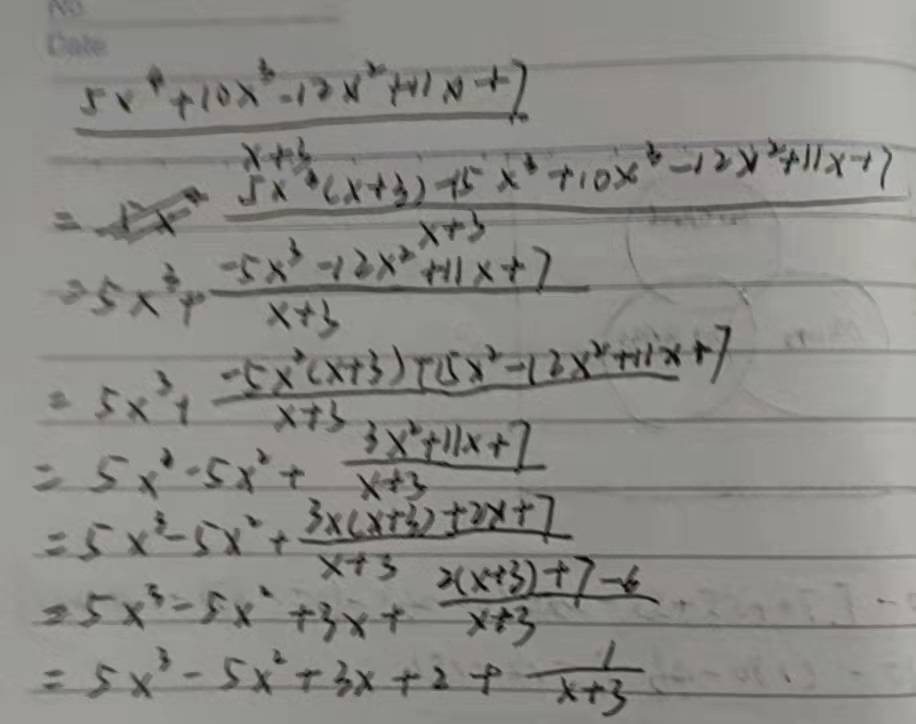### ¿Todavía tienes preguntas de matemáticas?

Pregunte a nuestros tutores expertos
Algebra
PreguntaUse synthetic division to find the quotient and remainder when $$5 x ^ { 4 } + 10 x ^ { 3 } - 12 x ^ { 2 } + 11 x + 7$$ is divided by $$x + 3$$ by completing the parts below.

(a) Complete this synthetic division table. (b) Write your answer in the following form: Quotient$$= \frac{Remainder}{x+ 3}$$

$$\frac { 5 x ^ { 4 } + 10 x ^ { 3 } - 12 x ^ { 2 } + 11 x + 7 } { x + 3 } =$$$$? + \frac { 1 } { x + 3 }$$